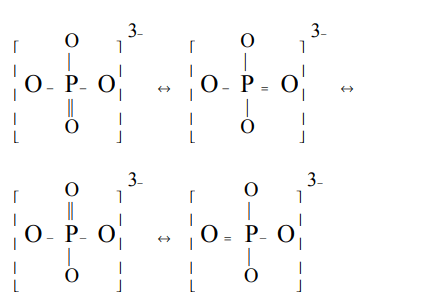## Chemical Bonding and Molecular Structure Questions and Answers Part-2

1. Ground state electronic configuration of valence shell electrons in nitrogen molecule (N2) is written as $\left(\sigma_{2s}\right)^{2}\left(\sigma*_{2s}\right)^{2}\left(\pi_{2p}\right)^{4}\left(\sigma_{2p}\right)^{2}$     Hence the bond order of nitrogen molecule is
a) 2
b) 3
c) 0
d) 1

Explanation:2. The bond order of NO molecule is
a) 1.5
b) 2.0
c) 2.5
d) 3.0

Explanation: Bond order in NO is 2.5

3. Which of the following cannot be formed ?
a) $He^{2+}$
b) $He^{+}$
c) He
d) $He_{2}$

Explanation: Bond order in $He_{2}$ is zero

4. $N_{2}$ and $N_{2}$ are converted into monoanions, $N_2^-$and $O_2^-$ respectively. Which of the following statements is wrong ?
a) In $N_2^-$ , N – N bond weakens
b) In $O_2^-$ , O - O bond order increases
c) In $O_2^-$ , O - O bond order decreases
d) $N_2^-$ becomes paramagnetic

Explanation:5. $N_{2}$ and $N_{2}$ are converted into monocations, $N_2^+$ and $O_2^+$ respectively. Which of the following statements is wrong?
a) In $N_2^+$ , N - N bond weakens
b) In $O_2^+$ , the O - O bond order increases
c) In $O_2^+$ , paramagnetism decreases
d) $N_2^+$ becomes diamagnetic

Explanation:6. Which of the following molecule is paramagnetic?
a) $CO_{2}$
b) $SO_{2}$
c) NO
d) $H_{2}O$

Explanation: NO is paramagnetic

7. In $PO_4^{3-}$ , the formal charge on each oxygen atom and the P - O bond order respectively are
a) –0.75, 0.6
b) –0.75, 1.0
c) –0.75, 1.25
d) –3, 1.25

Explanation:8. Which of the following ion has not bond order of 2.5 ?
a) $O_2^-$
b) $O_2^+$
c) $N_2^+$
d) $N_2^-$

Explanation: $O_2^-$ has Bond order 1.5

9. Oxygen molecule is
a) diamagnetic with no unpaired electrons
b) diamagnetic with two unpaired electrons
c) paramagnetic with two unpaired electrons
d) paramagnetic with no unpaired electrons